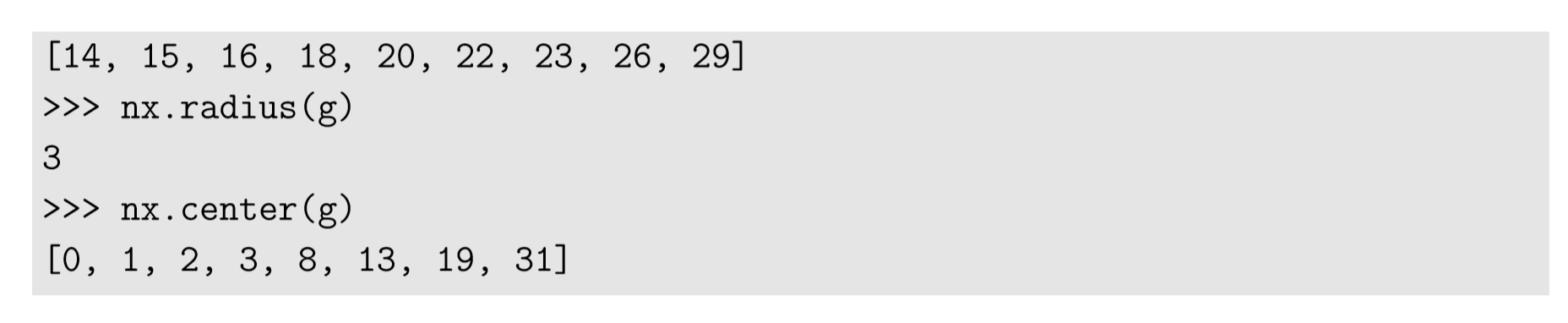Skip to main content
$$\newcommand{\id}{\mathrm{id}}$$ $$\newcommand{\Span}{\mathrm{span}}$$ $$\newcommand{\kernel}{\mathrm{null}\,}$$ $$\newcommand{\range}{\mathrm{range}\,}$$ $$\newcommand{\RealPart}{\mathrm{Re}}$$ $$\newcommand{\ImaginaryPart}{\mathrm{Im}}$$ $$\newcommand{\Argument}{\mathrm{Arg}}$$ $$\newcommand{\norm}{\| #1 \|}$$ $$\newcommand{\inner}{\langle #1, #2 \rangle}$$ $$\newcommand{\Span}{\mathrm{span}}$$

# 17.2: Shortest Path Length

$$\newcommand{\vecs}{\overset { \rightharpoonup} {\mathbf{#1}} }$$

$$\newcommand{\vecd}{\overset{-\!-\!\rightharpoonup}{\vphantom{a}\smash {#1}}}$$

Network analysis can measure and characterize various features of network topologies that go beyond size and density. Many of the tools used here are actually borrowed from social network analysis developed and used in sociology .

The ﬁrst measurement we are going to discuss is the shortest path length from one node to another node, also called geodesic distance in a graph. If the network is undirected, the distance between two nodes is the same, regardless of which node is the starting point and which is the end point. But if the network is directed, this is no longer the case in general.

The shortest path length is easily measurable using NetworkX:The actual path can also be obtained as follows:The output above is a list of nodes on the shortest path from node 16 to node 25. This can be visualized using draw_networkx_edges as follows:The result is shown in Fig. 17.2.1.Figure $$\PageIndex{1}$$: Visual output of Code 17.7.

We can use this shortest path length to deﬁne several useful metrics to characterize the network’s topological properties. Let’s denote a shortest path length from node $$i$$ to node $$j$$ as $$d(i → j)$$. Clearly, $$d(i → i) = 0$$ for all $$i$$. Then we can construct the following metrics:

Characteristic path length

$L =\frac{\sum_{i,j}{d(i \rightarrow{j})}}{n(n-1)} \label{(17.15)}$

where n is the number of nodes. This formula works for both undirected and directed networks. It calculates the average length of shortest paths for all possible node pairs in the network, giving an expected distance between two randomly chosen nodes. This is an intuitive characterization of how big (or small) the world represented by the network is.

Eccentricity
$\epsilon{(i) = \max_{j}{d(1 \rightarrow{j})}} \label{(17.16)}$

This metric is deﬁned for each node and gives the maximal shortest path length a node can have with any other node in the network. This tells how far the node is to the farthest point in the network.
Diameter

$D= \max_{i}{\epsilon(i)} \label{(17.17)}$

This metric gives the maximal eccentricity in the network. Intuitively, it tells us how far any two nodes can get from one another within the network. Nodes whose eccentricity is $$D$$ are called peripheries.
Radius

$R= \min_{i}{\epsilon{i}} \label{(17.18)}$

This metric gives the minimal eccentricity in the network. Intuitively, it tells us the smallest number of steps you will need to reach every node if you can choose an optimal node as a starting point. Nodes whose eccentricity is $$R$$ are called centers.

In NetworkX, these metrics can be calculated as follows:Exercise $$\PageIndex{1}$$

Visualize the Karate Club graph using the eccentricities of its nodes to color them.

Exercise $$\PageIndex{2}$$

Randomize the topology of the Karate Club graph while keeping its size and density, and then measure the characteristic path length, diameter, and radius of the randomized graph. Repeat this many times to obtain distributions of those metrics for randomized graphs. Then compare the distributions with the metrics of the original Karate Club graph. Discuss which metric is more sensitive to topological variations.

Note: Randomized graphs may be disconnected. If so, all of the metrics discussed above will be inﬁnite, and NetworkX will give you an error. In order to avoid this, you should check whether the randomized network is connected or not before calculating its metrics. NetworkX has a function nx.is_connected for this purpose.